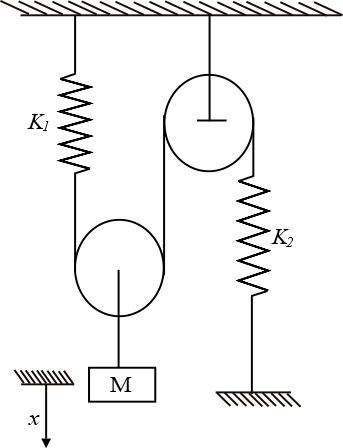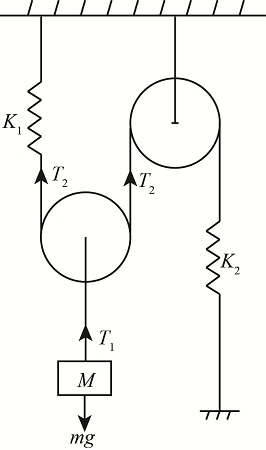# Consider the diagram below. All pulleys are massless and frictionless. We would like to find a...

## Question:

Consider the diagram below. All pulleys are massless and frictionless. We would like to find a simpler equivalent system for the motion of the mass (i.e., for the same {eq}x {/eq}), Find the equivalent spring, in terms of {eq}{{K}_{1}}\text{ and }{{K}_{2}} {/eq}.

HINT: Use the equivalent displacement under similar force method, the energy method will messy.## Spring Constant:

It is defined as the stiffness properties of spring. Spring constant is measured in Newton per meter and it is denoted by a term K. Spring constant is directly proportional to force and inversely proportional to displacement.

## Answer and Explanation:

Become a Study.com member to unlock this answer! Create your account

The following figure shows the free body diagram.The tension one due to weight of block is,

{eq}{T_1} = Mg {/eq}

Here,...

See full answer below.

#### Learn more about this topic:Practice Applying Spring Constant Formulas

from

Chapter 17 / Lesson 11
3.4K

In this lesson, you'll have the chance to practice using the spring constant formula. The lesson includes four problems of medium difficulty involving a variety of real-life applications.